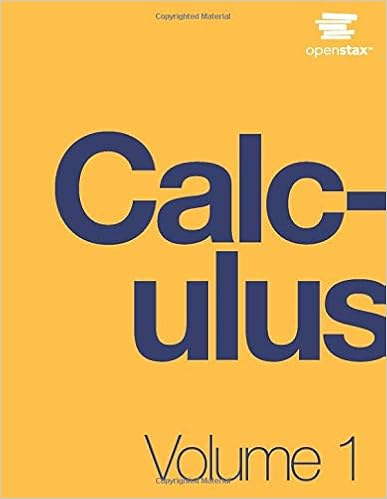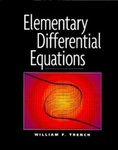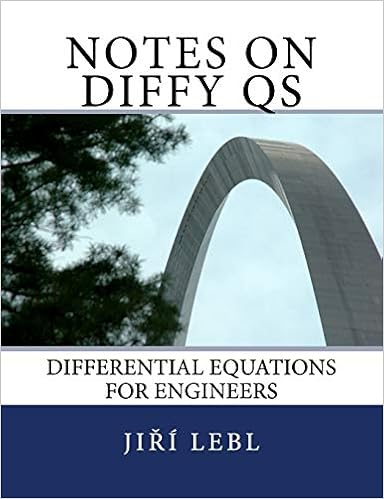# Mathematics

This guide is designed to assist faculty and students access scholarly Mathematics resources

#### PrecalculusJay Abramson, Valeree Falduto, et al., Precalculus

Precalculus is adaptable and designed to fit the needs of a variety of precalculus courses. It is a comprehensive text that covers more ground than a typical one- or two-semester college-level precalculus course. The content is organized by clearly-defined learning objectives, and includes worked examples that demonstrate problem-solving approaches in an accessible way.[PDF] Carl Stitz and Jeff Zeager, Precalculus

The authors are instructors at large community colleges in Ohio, and the book has now been adopted at several other locations. Those who have used the book expect to continue using it. One community college instructor now using it for the fourth time says he highly recommends the book to other colleagues and that his students often make positive comments about the book.

Approved by the American Institute of Mathematics.
Reviews available from the Open Textbook Library (the sections of this book titled College Algebra and College Trigonometry are also reviewed separately).David H. Collingwood, K. David Prince, and Matthew M. Conroy, Precalculus

The Mathematics Department of the University of Washington designed its precalculus to concentrate on two goals: 1) A review of the essential mathematics needed to succeed in calculus; 2) An emphasis on problem solving, the idea being to gain both experience and confidence in working with a particular set of mathematical tools. This text was written with those goals in mind. [...]

Approved by the American Institute of Mathematics.

#### CalculusDavid Guichard et al., Calculus: Early Transcendentals

The book covers the standard material in a calculus course for science and engineering. The size of the book is such that an instructor does not have to skip sections in order to fit the material into the typical course schedule. [...]

Approved by the American Institute of Mathematics.
Reviews available from the MAA, the Open Textbook Library, and BCcampusGilbert Strang, Edwin "Jed" Herman, et al., Calculus
Vol. 1 // Vol. 2 // Vol. 3

Calculus is designed for the typical two- or three-semester general calculus course, incorporating innovative features to enhance student learning. Volume 1 covers functions, limits, derivatives, and integration. Volume 2 covers integration, differential equations, sequences and series, and parametric equations and polar coordinates. Volume 3 covers parametric equations and polar coordinates, vectors, functions of several variables, multiple integration, and second-order differential equations.Matt Boelkins, David Austin, and Steven Schlicker, Active Calculus
Single Variable // Multivariable

Rather than detailed explanations and worked out examples, this book uses activities intended to be done by the students in order to present the standard concepts and computational techniques of calculus. The student activities provide most of the material to be assigned as homework, but since the book does not contain the usual routine exercises, instructors wanting such exercises will need to supply their own or use a homework system such as WebWork. [...]

Approved by the American Institute of Mathematics.
Reviews available from the Open Textbook Library.Michael Corral, Vector Calculus

The book has the material typically covered in the third semester of a mainstream calculus course for science, mathematics, and engineering students.

Approved by the American Institute of Mathematics.
Reviews available from SIAM Review.

#### Differential EquationsWilliam F. Trench, Elementary Differential Equations

This text is appropriate for a first course in differential equations for one or two semesters. There are more than 2000 exercises, and the student manual has solutions for most of the even numbered ones. A version with boundary value problems is also available.

Approved by the American Institute of Mathematics.
Reviews available from the Open Textbook Library.Jiří Lebl, Notes on Diffy Qs: Differential Equations for Engineers

This book is suitable for the typical one term course for science and engineering students that follows calculus. The book lends itself to a variety of course designs. [...]

Approved by the American Institute of Mathematics.

#### Linear AlgebraRob Beezer, A First Course in Linear Algebra

This book is a well-organized text with carefully constructed examples, a full quota of exercises with solutions, and an emphasis that is algebraic rather than geometric. The book is Sage-enabled with approximately 90 examples of Sage code spread throughout. [...]

Approved by the American Institute of Mathematics.
Reviews available from the MAA, SIAM Review, Notices of the AMS, and the Open Textbook Library.Jim Hefferon, Linear Algebra

This book has the standard content of a course for science, math, and engineering students that follows calculus. A semester of calculus is the explicit prerequisite, but most students would have three semesters of calculus and for them some of the beginning sections of the book can be skipped. [...]

Approved by the American Institute of Mathematics.
Reviews available from the MAAand the Open Textbook Library.Sergei Treil, Linear Algebra Done Wrong

Brown University has two introductory linear algebra courses. This text is used in the honors course that emphasizes proofs. The book’s title suggests that it is not the typical approach to linear algebra even among those books that are more theoretical. [...]

Approved by the American Institute of Mathematics.